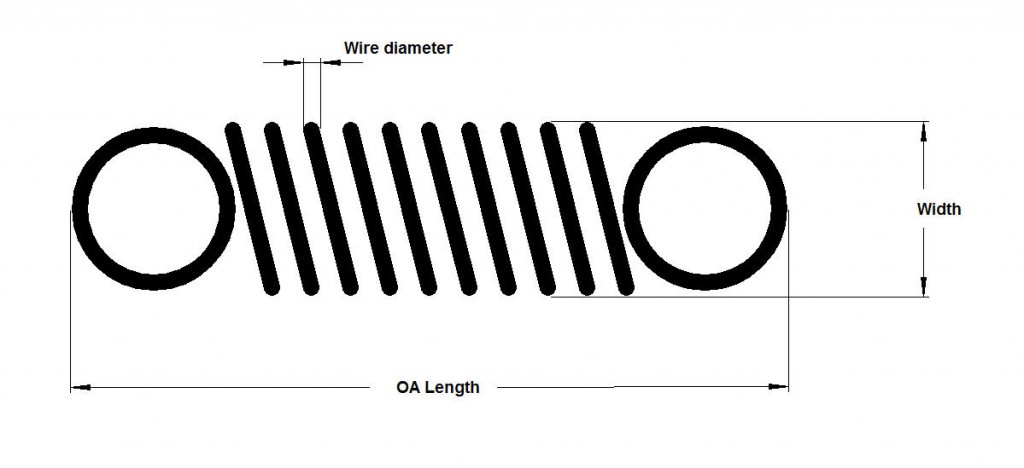# Spring data

## Explanation of ‘Rate’

#### For the techies-

K=Gd4 / (8nD3)
Where:
k = constant, pounds of load per unit of deflection
G = modulus of rigidity of spring material
d = wire diameter
n = number of active coils, which is the number of coils subjected to flexure (always less than the total number of coils)
D = mean coil diameter = Outer Diameter – Wire Diameter

G for stainless steel = 70300
G for carbon steel = 79300

Rate is the number of Newtons applied per mm of deflection
1″=24.4mm
1Kg=9.806N
1lb=4.448N
1Kg=2.205lb

## Measurements## Current stock items